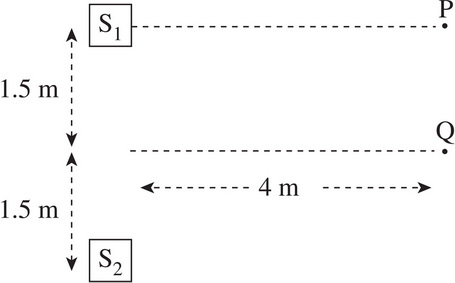# AP Physics 1: Waves Multiple-Choice Practice Questions

### Test Information10 questions18 minutes

1. What is the wavelength of a 5 Hz wave that travels with a speed of 10 m/s ?

2. A rope of length 5 m is stretched to a tension of 80 N. If its mass is 1 kg, at what speed would a 10 Hz transverse wave travel down the string?

3. A transverse wave on a long horizontal rope with a wavelength of 8 m travels at 2 m/s. At t = 0, a particular point on the rope has a vertical displacement of +A, where A is the amplitude of the wave. At what time will the vertical displacement of this same point on the rope be -A ?

4. A string, fixed at both ends, supports a standing wave with a total of 4 nodes. If the length of the string is 6 m, what is the wavelength of the wave?

5. A string, fixed at both ends, has a length of 6 m and supports a standing wave with a total of 4 nodes. If a transverse wave can travel at 40 m/s down the rope, what is the frequency of this standing wave?

6. A sound wave travels through a metal rod with wavelength λ and frequency f. Which of the following best describes the wave when it passes into the surrounding air?

Wavelength    Frequency

7. In the figure below, two speakers, S1 and S2, emit sound waves of wavelength 2 m, in phase with each other.Let AP be the amplitude of the resulting wave at Point P, and AQ the amplitude of the resultant wave at Point Q. How does AP compare to AQ?

8. An organ pipe that's closed at one end has a length of 17 cm. If the speed of sound through the air inside is 340 m/s, what is the pipe's fundamental frequency?

9. A bat emits a 40 kHz "chirp" with a wavelength of 8.75 mm toward a tree and receives an echo 0.4 s later. How far is the bat from the tree?

10. Two cars starting from the same position begin driving along the same horizontal axis. If Car A's horn emits a sound wave, which of the following pairs of velocities would result in the greatest difference between the wave's source frequency and the perceived frequency for Car B?

Car A    Car B